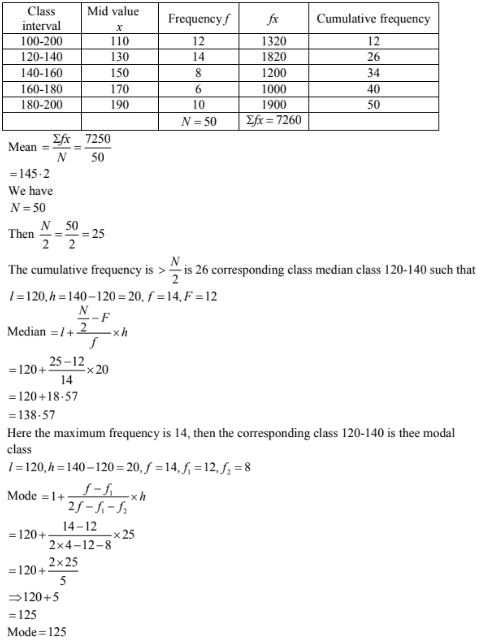# RD Sharma Solutions Chapter 7 Statistics Exercise 7.5 Class 10 Maths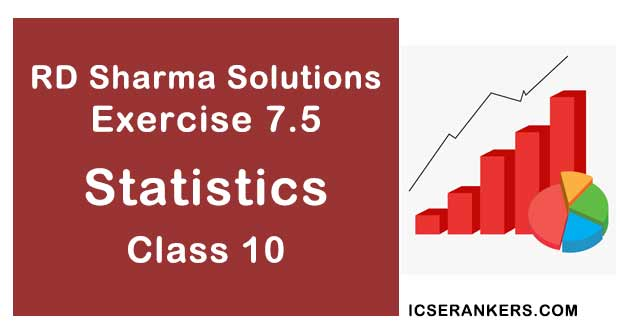Chapter Name RD Sharma Chapter 7 Statistics Book Name RD Sharma Mathematics for Class 10 Other Exercises Exercise 7.1Exercise 7.2Exercise 7.3Exercise 7.4Exercise 7.6 Related Study NCERT Solutions for Class 10 Maths

### Exercise 7.5 Solutions

1. Find the mode of the following data:
(i) 3,5,7,4,5,3,5,6,8,9,5,3,5,3,6,9,7,4
(ii) 3,3,7,4,5,3,5,6,8,9,5,3,5,3,6,9,7,4
(iii) 15,8,26,25,24,15,18,20,24,15,19,15

Solution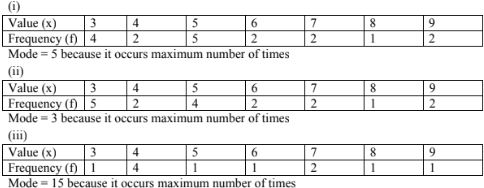2. The shirt sizes worn by a group of 200 persons, who bought the shirt from a store, are as follows:

 Shirt size: 37 38 39 40 41 42 43 44 Number of persons: 15 25 39 41 36 17 15 12

Find the model shirt size worn by the group.

Solution

 Shirt size: 37 38 39 40 41 42 43 44 Frequency (f) 15 25 39 41 36 17 15 12

Model shirt size = 40 because it occurs maximum number of times

3. Find the mode of the following distribution.

(i)

 Class interval: 0 – 10 10 – 20 20 – 30 30 – 40 40 – 50 50 – 60 60 – 70 70 – 80 Frequency: 5 8 7 12 28 20 10 10

(ii)

 Class – inteval: 10 – 15 15 – 20 20 – 25 25 – 30 30 – 35 35 – 40 Frequency: 30 45 75 35 25 15

(iii)

 Class – interval: 25 – 30 30 – 35 35 – 40 40 – 45 45 – 50 50 – 60 Frequency: 25 34 50 42 38 14

Solution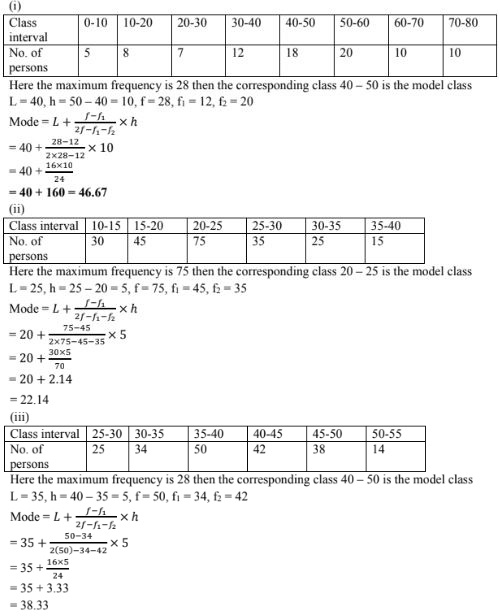4. Compare the modal ages of two groups of students appearing for an entrance test:
 Age(in years): 16 – 18 18 – 20 20 – 22 22 – 24 24 – 26 Group A 50 78 46 28 23 Group B 54 89 40 25 17

Solution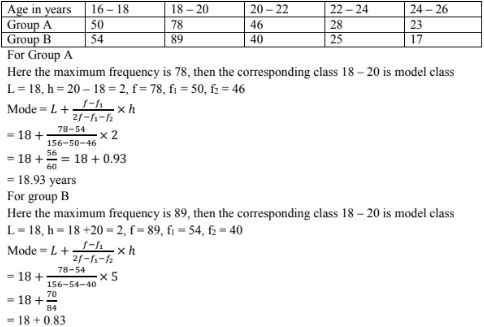= 18.83
Hence the mode of age for the group A is higher than group B.

5. The marks in science of 80 students of class X are given below: Find the mode of the marks obtained by the students in science.
 Marks: 0 – 10 10 – 20 20 – 30 30 – 40 40 – 50 50 – 60 60 – 70 70 – 80 80 – 90 90 – 100 Frequency: 3 5 16 12 13 20 5 4 1 1

Solution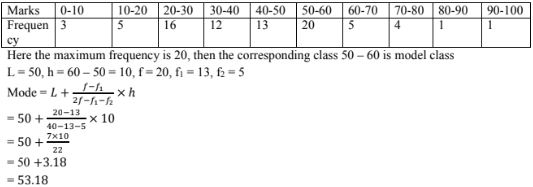6. The following is the distribution of height of students of a certain class in a certain city:
 Height(in cm): 160 – 162 163 – 165 166 – 168 169 – 171 172 – 174 No. of students: 15 118 142 127 18

Find the average height of maximum number of students.

Solution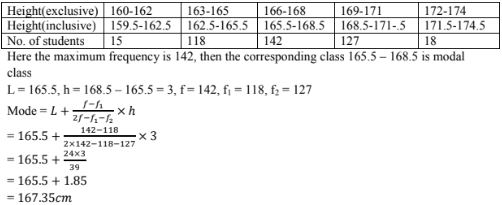7. The following table shows the ages of the patients admitted in a hospital during a year:
 Age(in years): 5 – 15 15 – 25 25 – 35 35 – 45 45 – 55 55- 65 No. of students: 6 11 21 23 14 5

Find the mode and the mean of the data given above. Compare and interpret the two measures of central tendency.

Solution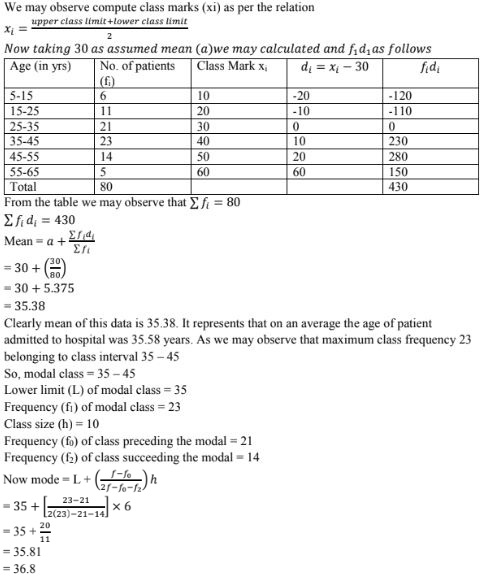8. The following data gives the information on the observed lifetimes (in hours) of 225 electrical components:
 Lifetimes (in hours): 0 – 20 20 – 40 40 – 60 60 – 80 80 – 100 100 – 120 No. of components: 10 35 52 61 38 29

Determine the modal lifetimes of the components.

Solution
From data as given above we may observe that maximum class frequency 61 belonging to
class interval 60 - 80.
So, modal class 60 - 80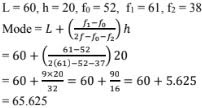9. The following data gives the distribution of total monthly houshold expenditure of 200 families of a village. Find the modal monthly expenditure of the families.Also, find the mean monthly expenditure:
 Expenditure (in Rs.) Frequency Expenditure(in Rs.) Frequency 1000 – 1500 24 3000 – 3500 30 1500 – 2000 40 3500 – 4000 22 2000 – 2500 33 4000 – 4500 16 2500 – 3000 28 4500 – 5000 7

Solution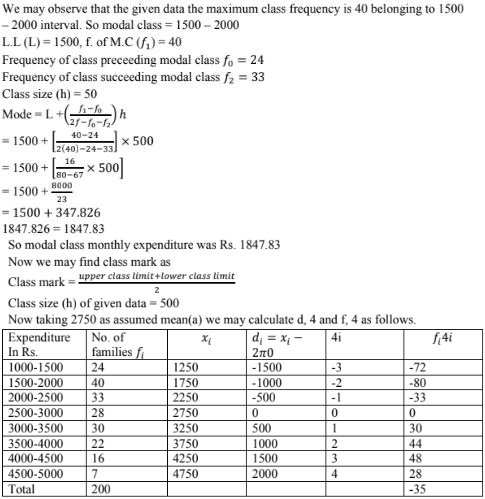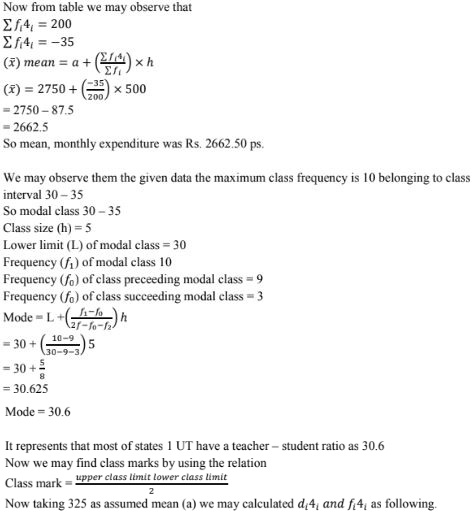10. The following distribution gives the state – wise teacher – student ratio in higher secondary schools of India. Find the mode and mean of this data. Interpret, the two measures:
 Number of students per teacher Number of states/U.T. Number of students per teacher Number of states/U.T. 15 – 20 3 35 – 40 3 20 – 25 8 40 – 45 0 25 – 30 9 45 – 50 0 30 – 35 10 50 – 55 2

Solution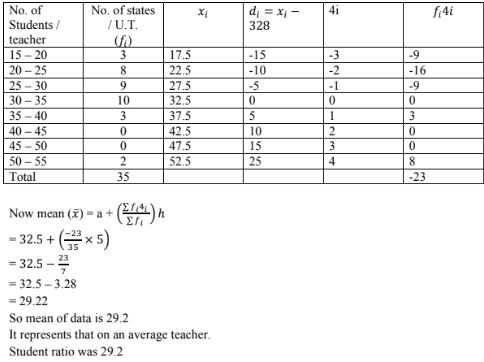11. The given distribution shows the number of runs scored by some top batsmen of the world in one – day international cricket matches.
 Runs scored No. of batsman Runs scored No. of batsman 3000 – 4000 4 7000 – 8000 6 4000 – 5000 18 8000 – 9000 3 5000 – 6000 9 9000 – 10000 1 6000 – 7000 7 10000 – 11000 1

Find the mode of the data.

Solution

From the given data we may observe that maximum class frequently is 18 belonging to
class interval  4000 - 5000
So modal class 4000 - 5000
Lower limit(1) of model class = 4000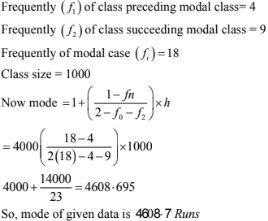12. A student noted the number of cars passing through a spot on a road for loo periods each of 3 minutes and summarized it in the table given below. Find the mode of the data:

Solution

From the given data we may observe that maximum class internal frequency is 200 belonging to modal class = 40 - 50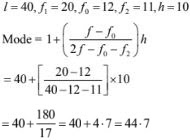13. The following frequency distribution gives the monthly consumption of electricity of 68 consumers of a locality. Find the median, mean and mode of the data the compare them.
 Monthly consumption(in units) 65 – 85 85 – 105 105 – 125 125 – 145 145 – 165 165 – 185 185 – 205 No. of consumers: 4 5 13 20 14 8 4

Solution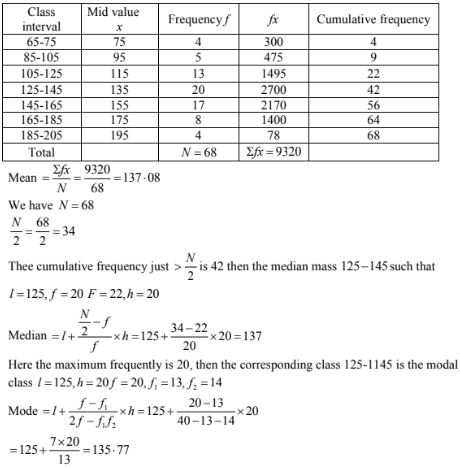14. 100 surnames were randomly picked up from a local telepone directly and the frequency distribution of the number of letters in the English alphabets in the surnames was obtained as follows:
 Number of letters: 1 – 4 4 – 7 7 – 10 10 – 13 13 – 16 16 – 19 Number surnames: 6 30 40 16 4 4

Determine the median number of letters in the surnames. Find the mean number of letters in the surnames. Also, find the modal size of the surnames.

Solution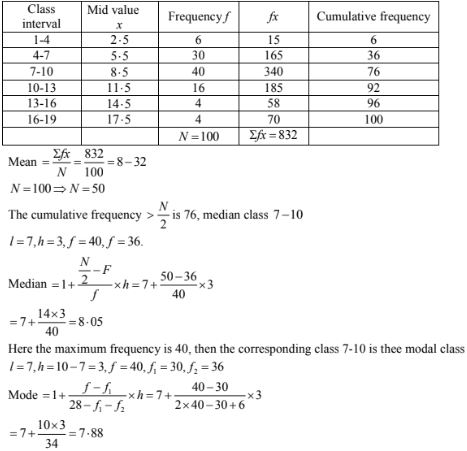15. Find the mean, median and mode of the following data:
 Classes: 0 – 20 20 – 40 40 – 60 60 – 80 80 – 100 100 – 120 120 – 140 Frequency: 6 8 10 12 6 5 3

Solution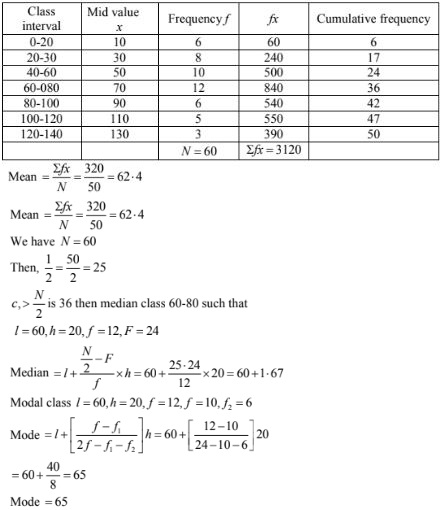16. Find the mean, median and mode of the following data:
 Classes : 0 – 50 50 – 100 100 – 150 150 – 200 200 – 250 250 – 300 300 – 350 Frequency: 2 3 5 6 5 3 1

Solution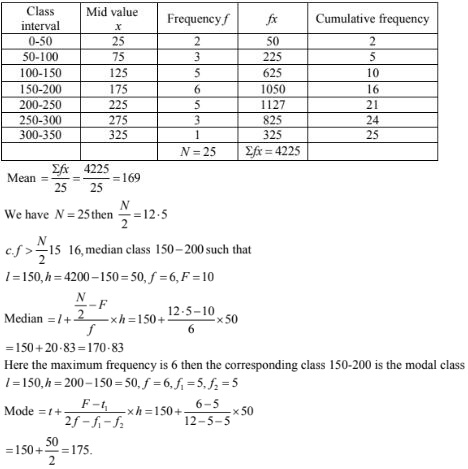17. The following table gives the daily income of 50 workers of a factory:
 Daily income(in Rs) 100 – 120 120 – 140 140 – 160 160 – 180 180 – 200 Number of workers: 12 14 8 6 10

Find the mean, mode and median of the above data.

Solution